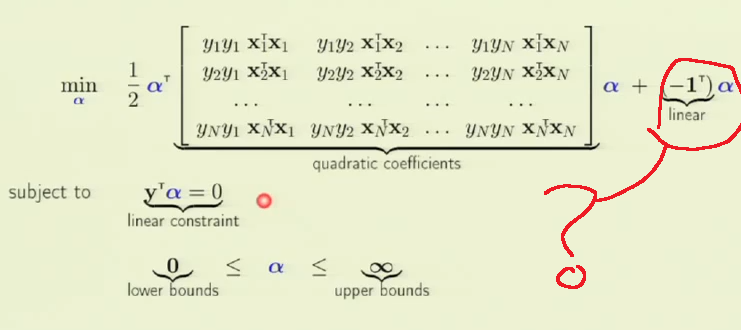LFD Book ForumQuadratic programming
 Register FAQ Calendar Mark Forums Read#1
 invisSenior Member Join Date: Jul 2012 Posts: 50Quadratic programming

I never used QP in my practice. And I using Octave for solve HWs.
So there is built-in function for quadratic programming in Octave - qp. (example)

But I still dont know what parameters I should use to solve:s.t.Looks like H is(.* is element by element product)
But what is the other parameters#2
 invisSenior Member Join Date: Jul 2012 Posts: 50Re: Quadratic programming

And where is ?#3
 invisSenior Member Join Date: Jul 2012 Posts: 50Re: Quadratic programming

I am absolutly confusedShould I use QP to solve this inequality:s.t.OR to solve inequality in picture in previous message ?
#4yaserCaltech Join Date: Aug 2009 Location: Pasadena, California, USA Posts: 1,478Re: Quadratic programming

Quote:
 Originally Posted by invisI am absolutly confusedShould I use QP to solve this inequality:s.t.OR to solve inequality in picture in previous message ?
The version in the picture. It's the dual problem (equivalent) to the version that is in the quote.
__________________
Where everyone thinks alike, no one thinks very much
#5
 invisSenior Member Join Date: Jul 2012 Posts: 50Re: Quadratic programming

Why sometimes it is impossible to find the solution and what to do with that ?
On 200 iterations with random line and random dots (#10) 81 times QP didnt find the solution (I plot the line and dots for this situations and all looks pretty clear).
This is the parameters for QP I use:

H = (Y*Y') .* (X*X');
A = Y';
q=-1*ones(n,1);
b=0;
lb=zeros(n,1); (lower bound)
ub=[]; (upper bound)

min 0.5 x'*H*x + x'*q
subject to

A*x = b
lb <= x <= ub

where x is our#6htlinNTU Join Date: Aug 2009 Location: Taipei, Taiwan Posts: 601Re: Quadratic programming

Quote:
 Originally Posted by invisWhy sometimes it is impossible to find the solution and what to do with that ? On 200 iterations with random line and random dots (#10) 81 times QP didnt find the solution (I plot the line and dots for this situations and all looks pretty clear). This is the parameters for QP I use: H = (Y*Y') .* (X*X'); A = Y'; q=-1*ones(n,1); b=0; lb=zeros(n,1); (lower bound) ub=[]; (upper bound) min 0.5 x'*H*x + x'*q subject to A*x = b lb <= x <= ub where x is ourSometimes the numerical condition of the optimization problem makes it hard for the solver to locate a good solution. One possibility is to set ub (upper bound) to a really large value. Hope this helps.
__________________
When one teaches, two learn.
#7
 invisSenior Member Join Date: Jul 2012 Posts: 50Re: Quadratic programming

I change ub to:
ub=10^22*ones(n,1);

For 400 iterations 154 without solutionHow to deal with it in context of homework ?
"How often isbetter thanin approximating?"
#8
 jakvasMember Join Date: Jul 2012 Posts: 17Re: Quadratic programming

@invis

for the 100 points problem 400 iterations may be too little (i used 2000 iterations in my matlab code and in ~2% of the cases even that limit was exceeded but that still gives a decent accuracy you could use even more but don't go too far or you will never get results) also for the upper bound I used 10^5 and 10^10 without any significant change in results, 10^22 seems a bit much considering you are probably using single precision numbers#9
 invisSenior Member Join Date: Jul 2012 Posts: 50Re: Quadratic programming

400 iterations I use only to show that ~40% of problems QP cant solve even with 10 dots. So how can I compare results with PLA ?

Jakvas you are using matlab, so maybe you can tell me am I miss something in parameters for QP ? Why almost half of problems without solution ?
#10
 jakvasMember Join Date: Jul 2012 Posts: 17Re: Quadratic programming

try a smaller upper bound and maybe plot some of the results you do get to see if there is no serious error somewhere.Tags quadratic programmingThread ToolsShow Printable VersionEmail this Page Display ModesLinear ModeSwitch to Hybrid ModeSwitch to Threaded ModePosting Rules You may not post new threads You may not post replies You may not post attachments You may not edit your posts BB code is On Smilies are On [IMG] code is On HTML code is Off Forum Rules
 Forum Jump User Control Panel Private Messages Subscriptions Who's Online Search Forums Forums Home General     General Discussion of Machine Learning     Free Additional Material         Dynamic e-Chapters         Dynamic e-Appendices Course Discussions     Online LFD course         General comments on the course         Homework 1         Homework 2         Homework 3         Homework 4         Homework 5         Homework 6         Homework 7         Homework 8         The Final         Create New Homework Problems Book Feedback - Learning From Data     General comments on the book     Chapter 1 - The Learning Problem     Chapter 2 - Training versus Testing     Chapter 3 - The Linear Model     Chapter 4 - Overfitting     Chapter 5 - Three Learning Principles     e-Chapter 6 - Similarity Based Methods     e-Chapter 7 - Neural Networks     e-Chapter 8 - Support Vector Machines     e-Chapter 9 - Learning Aides     Appendix and Notation     e-Appendices

All times are GMT -7. The time now is 06:38 PM.